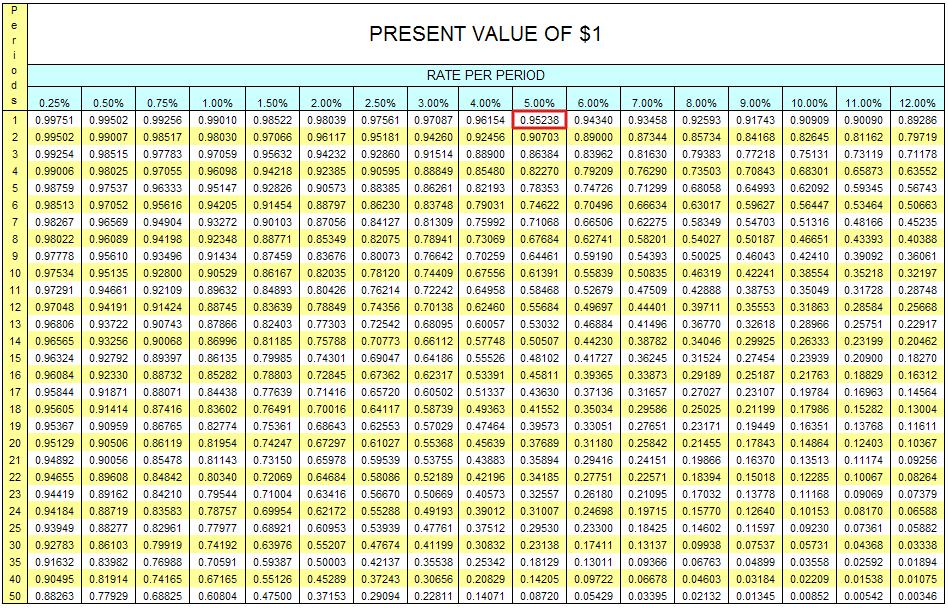# What is a Present Value Table?

Definition: A present value table is a tool that helps analysts calculate the PV of an amount of money by multiplying it by a coefficient found on the table. In other words, it is a table that illustrates the different coefficients that can be used to calculate a figure’s present value depending on the discount rate and period of time used.

## What Does Present Value Table Mean?

What is the definition of present value table? A PV table lists different discount rates in the first column and different time periods in the first row. The purpose of the table is to provide present value coefficients for different time periods and discount rates. Periods can be presented in weeks, months or years and discount rates normally go from 0 to 20% with intervals of 0.25% or 0.50% between them.

These tables eliminate the need for a financial calculator or the requirement to do long calculations by hand, but they are not as accurate a using the actual equation or a financial calculator. The coefficients in the table typically rounded to the fourth decimal place. Thus, there tend to be rounding errors in the calculations as well.

Let’s take a look at an example.

## Example

A company is evaluating a business deal to sell a given asset. The current market price of the asset is \$5,000. The person interested in buying it is offering to pay \$7,000 for the asset and the payment will be made in a year. The company needs to evaluate if the current present value of that offer is higher than the \$5,000 price to assess the profitability of the deal. The company’s current discount rate is 5%.

By using a PV table, the company can identify the coefficient that should be multiplied by the \$7,000 figure to get its PV. The coefficient for a transaction settled in a year at a discount rate of 5% is 0.95238.By multiplying \$7,000 by this coefficient, we get a PV of \$6,666.66, which is far superior to the \$5,000 price the company is expecting. This means the deal should be accepted because it will be profitable for the company.

## Summary Definition

Define Present Value Table: PV table means a chart used to calculate present values of numbers without using a financial calculator.